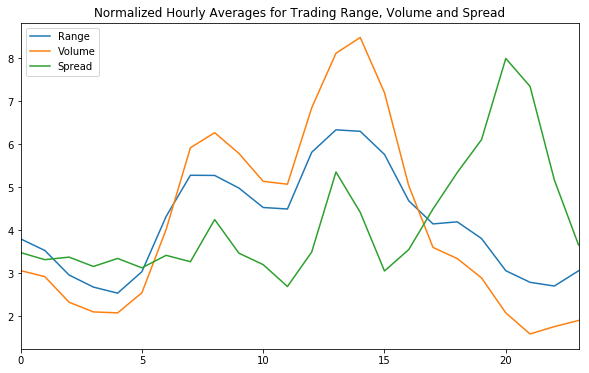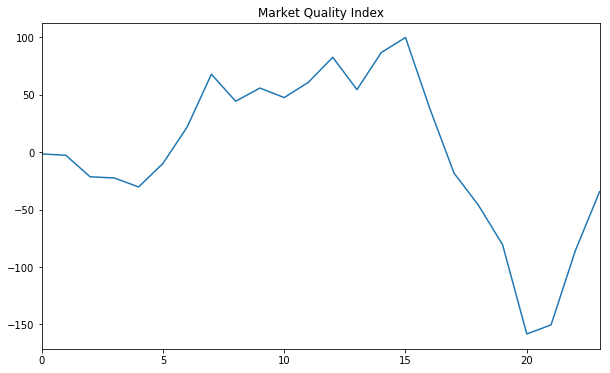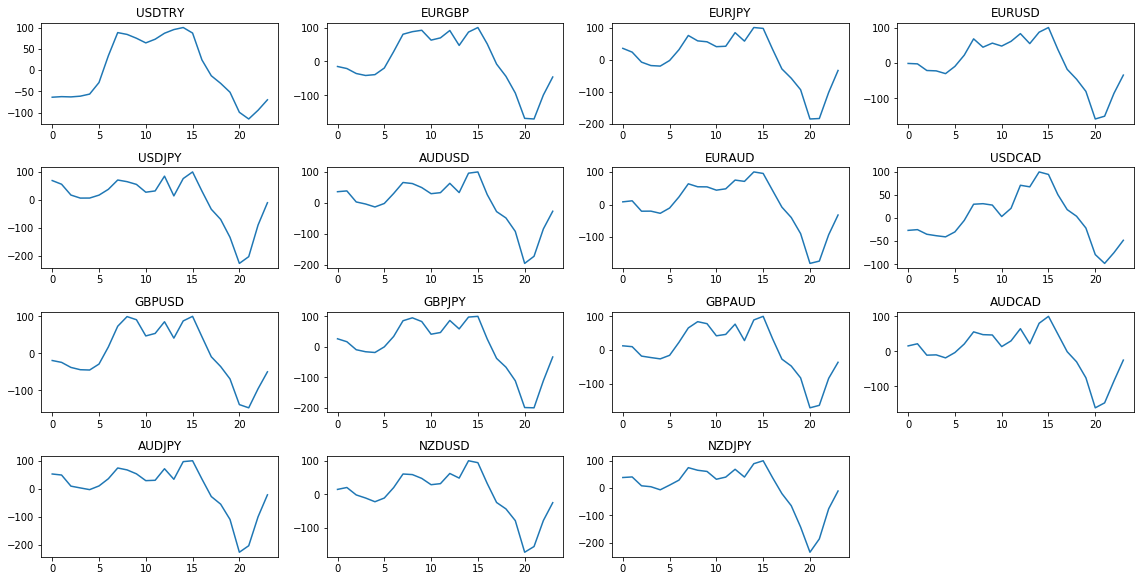### Time of Day effects in FX

Time of day is critical for trading, it is even possible building trading strategies solely depending on time of day (I will keep this for another post)

I will be using the concept of quality and define a high quality market, from an intraday timing perspective, as a market when trading range and volume are high and spread is low. I assume this as a good time to trade as trading cost (spread) is low compared to trading opportunity (range and volume)

I start importing libraries,

In :
```import matplotlib.pyplot as plt
import pandas as pd
import bulk_tester as bt
import numpy as np
%matplotlib inline
```

I then load data for a couple of years for EURUSD, here I am using my own library for loading it from a csv file containing 15 minute bars and sampling at each 60 minutes.

In :
```pair = "EURUSD"
dftest = bt.get_data(pair, 'BT', resample_period='60T')
```

Then I add three columns; hour for doing the hourly grouping, spread and range

In :
```dftest['hour'] = dftest.index.hour
```

I use 3 pivot tables for caclulating and grouping volume, spread and range by hour

In :
```#find hourly mean volume
table_volume = pd.pivot_table(dftest, values='volume', index=['hour'],
aggfunc=np.mean)
```
In :
```#find hourly mean spread
aggfunc=np.mean)
```
In :
```#find hourly mean range
table_range = pd.pivot_table(dftest, values='range', index=['hour'],
aggfunc=np.mean)
```

I then create a new dataframe with normalized values for range, volume and spread so that they can fit into the same vertical range in a plot. Note that these are not the actual values!

In :
```summary=pd.DataFrame()
summary["Range"] = table_range/sum(table_range.values)*100
summary["Volume"] = table_volume/sum(table_volume.values)*100
```
In :
```title = "Normalized Hourly Averages for Trading Range, Volume and Spread"
summary.plot(kind='line', figsize = (10,6), title=title)
```
Out:
`<matplotlib.axes._subplots.AxesSubplot at 0x10b399668>`Looking at trading GMT hours for FX sessions:

Australia = [20,21,22,23,0,1,2,3,4]

Tokyo = [0,1,2,3,4,5,6,7,8]

Europe = [8,9,10,11,12,13,14,15,16]

Americas = [13,14,15,16,17,18,19,20,21]

it can be seen that average volume and range peaks at around US open and spread is close to its minumum at 15:00

In line with my definition of market quality and considering we have normalized figures, I calculate market quality as:

(Volume + Range) / 2 - Spread

and calculate a market quality index (MQI) which peaks at 100 indicating that the opportunity-cost gap is at maximum.

In :
```summary["Quality"] = (((summary.Volume)+(summary.Range))/2-(summary.Spread))
mqi = summary.Quality*100/summary.Quality.max()
mqi.plot(kind="line", figsize = (10,6), title='Market Quality Index')
```
Out:
`<matplotlib.axes._subplots.AxesSubplot at 0x10c001e80>`MQI peaks at 12:00 - 15:00 which is the overlap of Europe and US sessions. MQI bottoms at 20:00 before US session closing. Tokyo and Australia sessions are low range, low volume and high spread sessions

Putting all this together and checking for a set of pairs... an obvious pattern. So what...For intraday traders holding positions for a couple of hours, executing trades when MQI is at its peaks is a good idea as it maximizes the opportunity to cost gap. And it is important to note that despite the similarities, MQI pattern is not the same for all pairs. As an example, looking at USDCAD, MQI peaks in a relatively narrow range and Europe session does not contribute much as it does to other pairs.

In :
```plt.figure(1, figsize=(16, 30))

#get a list of pair names to loop

a=0#counter for plotting

#loop a list of pairs and plot MQI
for pair in df.PAIRNAME:

s="";
pair = s.join([pair[:3], pair[4:7]])

dftest = bt.get_data(pair, 'BT', resample_period='60T')
dftest['hour'] = dftest.index.hour
#find hourly mean volume
table_volume = pd.pivot_table(dftest, values='volume', index=['hour'],
aggfunc=np.mean)
aggfunc=np.mean)
#find hourly mean range
table_range = pd.pivot_table(dftest, values='range', index=['hour'],
aggfunc=np.mean)
summary=pd.DataFrame()
summary["Range"] = table_range/sum(table_range.values)*100
summary["Volume"] = table_volume/sum(table_volume.values)*100
mqi = summary.Quality*100/summary.Quality.max()

a+=1#counter for plotting

plt.subplot(len(df),4,a)
plt.plot(mqi)
plt.yscale('linear')
plt.title(pair)
plt.grid(False)
plt.tight_layout()
```In [ ]:
```
```

1.Language sorry i am little dummie and beginner

1.I will be happy to answer if you have a question.

2.Nice information. Forex trading is a very profitable and very risky business opportunity. With good strategy you can make more profit.

3.i was once living on the street where by things were so hard for me, even to pay off my bills was very difficult for me i have to park off my apartment and start sleeping on the street of Vegas. i tried all i could do to secure a job but all went in vain because i was from the black side of America. so i decided to browse through on my phone for jobs online where i got an advert on Hackers advertising a Blank ATM card which can be used to hack any ATM Machine all over the world, i never thought this could be real because most advert on the internet are based on fraud, so i decided to give this a try and look where it will lead me to if it can change my life for good. i contacted this hackers and they told me they are from Australia and also they have branch all over the world in which they use in developing there ATM CARDS, this men who were geeks and also experts at ATM repairs, programming and execution who taught me various tips and tricks about breaking into an ATM Machine with a Blank ATM card.i applied for the Blank ATM card and it was delivered to me within 3 days and i did as i was told to and today my life has changed. there is no ATM MACHINES this BLANK ATM CARD CANNOT penetrate into, because it has been programmed with various tools and software before it will be send to you. i know this is illegal but also a smart way of living Big because the government cannot help us so we have to help our self. if you also want this BLANK ATM CARD you can contact these Hackers at